1626083151

Text Classification with TensorFlow Keras, NLP Using Embedding and LSTM Recurrent Neural Networks

In this video I’m creating a baseline NLP model for Text Classification with the help of Embedding and LSTM layers from TensorFlow’s high-level API Keras.

00:00 NLP with TensorFlow
00:48 How to clean text data for machine learning
01:56 How to count the occurences of each word in a corpus
03:40 Why we need to define the sequence length for NLP Projects with Tensorflow
04:00 How to split the dataset into a train and test set
04:42 How to use Tokenizer from Keras to index words and transform text to sequences
05:49 How to pad text sequences to have a specific length for NLP Projects with Tensorflow
08:15 LSTM Model for NLP Projects with Tensorflow
08:25 Understanding Embedding and why we need to use it for NLP Projects

With Embedding, we map each word to a vector of fixed size with real-valued elements. In contrast to one hot encoding, we can use finite sized vectors to represent an infinite number of real numbers.

This feature learning technique can learn the most important features to represent the words in the data.

LSTMs are Recurrent Neural Networks (RNN) used for modeling sequences. LSTM units have a memory cell as the building block and it represents the hidden layer. In an LSTM cell there are three different types of gates: the forget gate, the input gate and the output gate.

The most important one, the forget gate allows the LSTM memory cell to reset the cell state. The forget gate decides which information is allowed to go through and which to hold back.

You can access the Jupyter notebook here (login required):

https://www.decisionforest.com/downlo…

#python #nlp #tensorflow

Buddha Community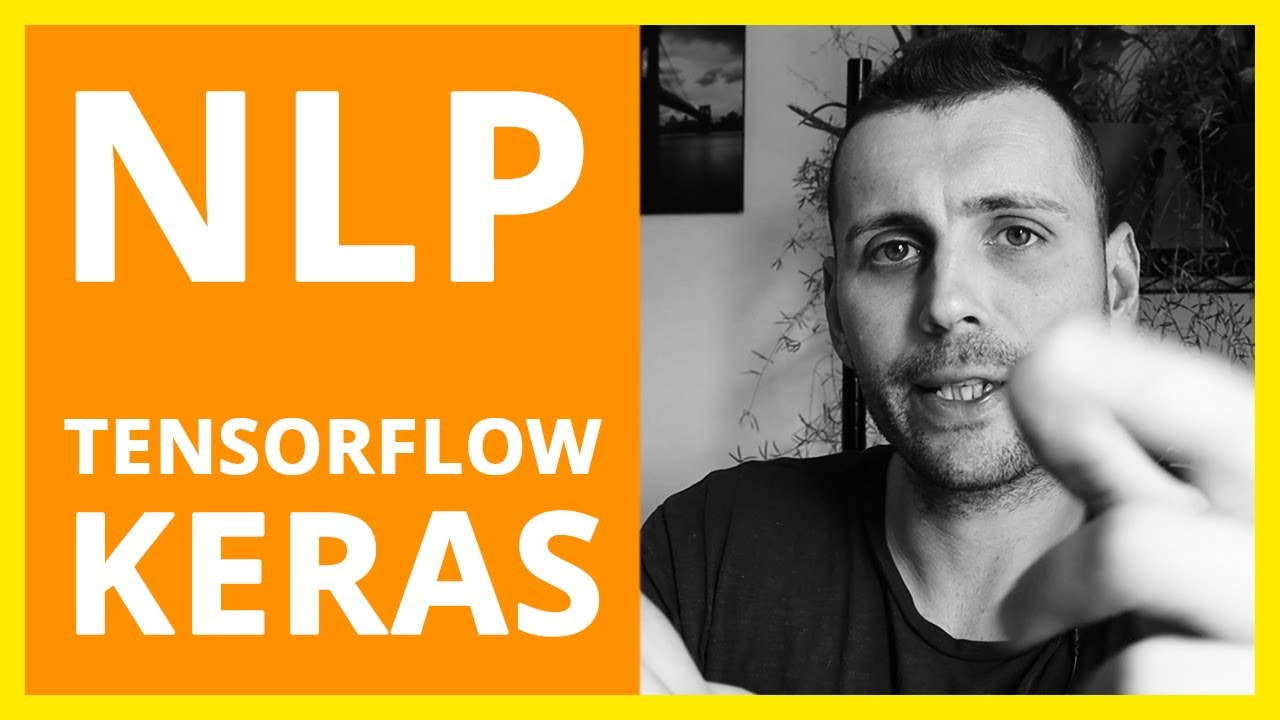1622273248

Sentiment Analysis Using TensorFlow Keras - Analytics India Magazine

Natural Language Processing is one of the artificial intelligence tasks performed with natural languages. The word ‘natural’ refers to the languages that evolved naturally among humans for communication. A long-standing goal in artificial intelligence is to make a machine effectively communicate with humans. Language modeling and Language generation (such as neural machine translation) have been popular among researchers for over a decade. For an AI beginner, learning and practicing Natural Language Processing can be initialized with classification of texts. Sentiment Analysis is among the text classification applications in which a given text is classified into a positive class or a negative class (sometimes, a neutral class, too) based on the context. This article discusses sentiment analysis using TensorFlow Keras with the IMDB movie reviews dataset, one of the famous Sentiment Analysis datasets.

TensorFlow’s Keras API offers the complete functionality required to build and execute a deep learning model. This article assumes that the reader is familiar with the basics of deep learning and Recurrent Neural Networks (RNNs). Nevertheless, the following articles may yield a good understanding of deep learning and RNNs:

#developers corner #imdb dataset #keras #lstm #lstm recurrent neural network #natural language processing #nlp #recurrent neural network #rnn #sentiment analysis #sentiment analysis nlp #tensorflow1626083151

Text Classification with TensorFlow Keras, NLP Using Embedding and LSTM Recurrent Neural Networks

In this video I’m creating a baseline NLP model for Text Classification with the help of Embedding and LSTM layers from TensorFlow’s high-level API Keras.

00:00 NLP with TensorFlow
00:48 How to clean text data for machine learning
01:56 How to count the occurences of each word in a corpus
03:40 Why we need to define the sequence length for NLP Projects with Tensorflow
04:00 How to split the dataset into a train and test set
04:42 How to use Tokenizer from Keras to index words and transform text to sequences
05:49 How to pad text sequences to have a specific length for NLP Projects with Tensorflow
08:15 LSTM Model for NLP Projects with Tensorflow
08:25 Understanding Embedding and why we need to use it for NLP Projects

With Embedding, we map each word to a vector of fixed size with real-valued elements. In contrast to one hot encoding, we can use finite sized vectors to represent an infinite number of real numbers.

This feature learning technique can learn the most important features to represent the words in the data.

LSTMs are Recurrent Neural Networks (RNN) used for modeling sequences. LSTM units have a memory cell as the building block and it represents the hidden layer. In an LSTM cell there are three different types of gates: the forget gate, the input gate and the output gate.

The most important one, the forget gate allows the LSTM memory cell to reset the cell state. The forget gate decides which information is allowed to go through and which to hold back.

You can access the Jupyter notebook here (login required):

https://www.decisionforest.com/downlo…

#python #nlp #tensorflow1594366200

Recurrent Neural Networks for Multilabel Text Classification Tasks

The purpose of this project is to build and evaluate Recurrent Neural Networks(RNNs) for sentence-level classification tasks. I evaluate three architectures: a two-layer Long Short-Term Memory Network(LSTM), a two-layer Bidirectional Long Short-Term Memory Network(BiLSTM), and a two-layer BiLSTM with a word-level attention layer. Although they do learn useful vector representation, BiLSTM with attention mechanism focuses on necessary tokens when learning text representation. To that end, I’m using the 2019 Google Jigsaw published dataset on Kaggle labeled “Jigsaw Unintended Bias in Toxicity Classification.” The dataset includes 1,804,874 user comments, with the toxicity level being between 0 and 1. The final models can be used for filtering online posts and comments, social media policing, and user education.

Recurrent Neural Networks Overview

RNNs are neural networks used for problems that require sequential data processing. For instance:

• In a sentiment analysis task, a text’s sentiment can be inferred from a sequence of words or characters.
• In a stock prediction task, current stock prices can be inferred from a sequence of past stock prices.

At each time step of the input sequence, RNNs compute the output yt and an internal state update ht using the input xt and the previous hidden-state ht-1. They then pass information about the current time step of the network to the next. The hidden-state ht summarizes the task-relevant aspect of the past sequence of the input up to t, allowing for information to persist over time.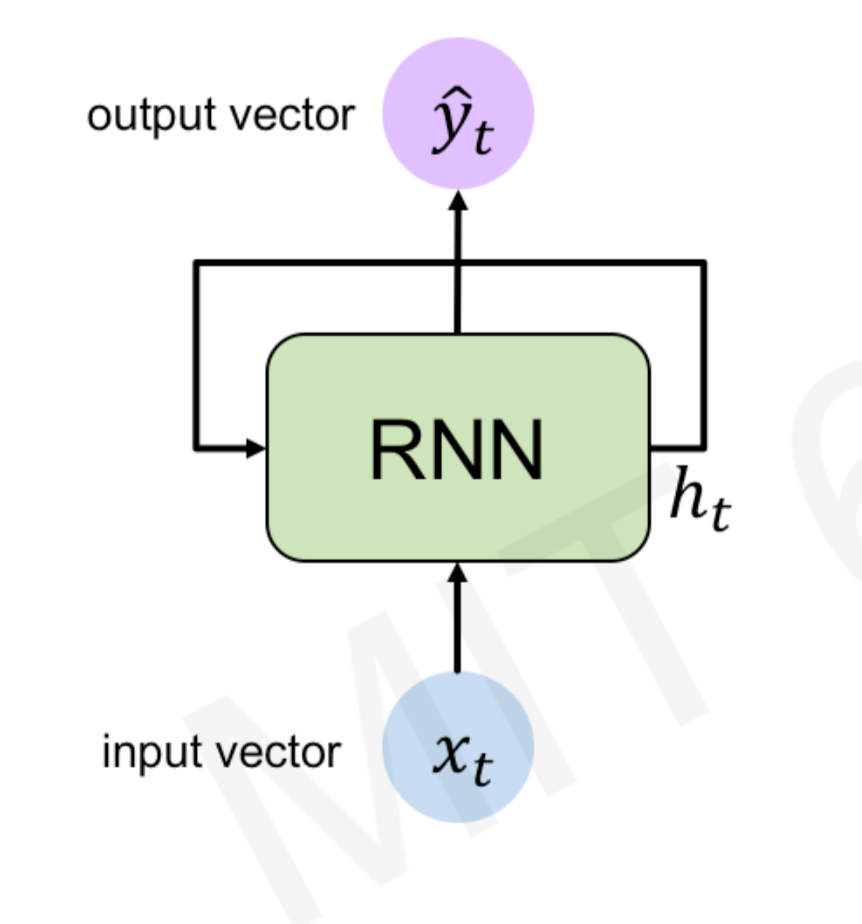Recurrent Neural Network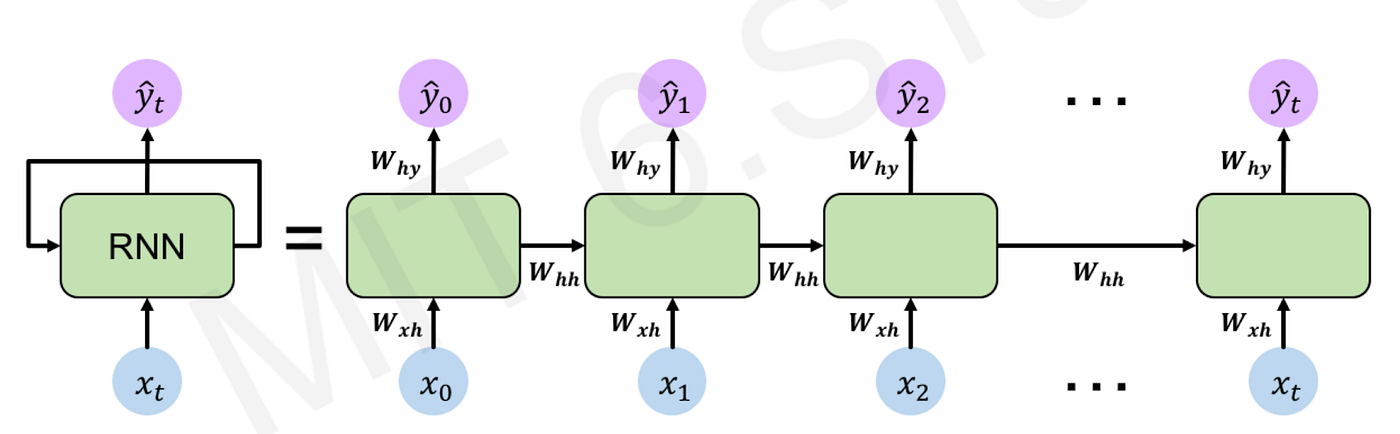Recurrent Neural Network

During training, RNNs re-use the same weight matrices at each time step. Parameter sharing enables the network to generalize to different sequence lengths. The total loss is a sum of all losses at each time step, the gradients with respect to the weights are the sum of the gradients at each time step, and the parameters are updated to minimize the loss function.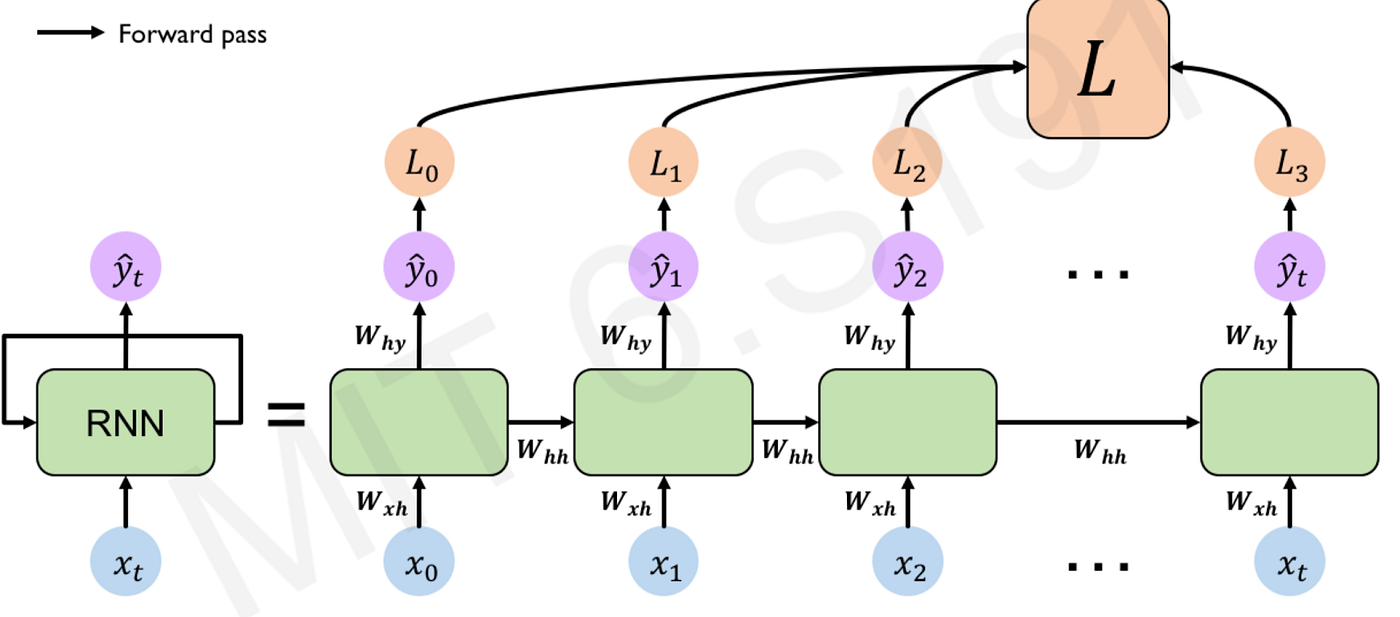forward pass: compute the loss function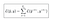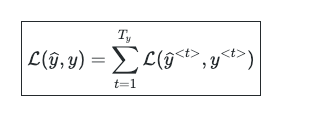loss function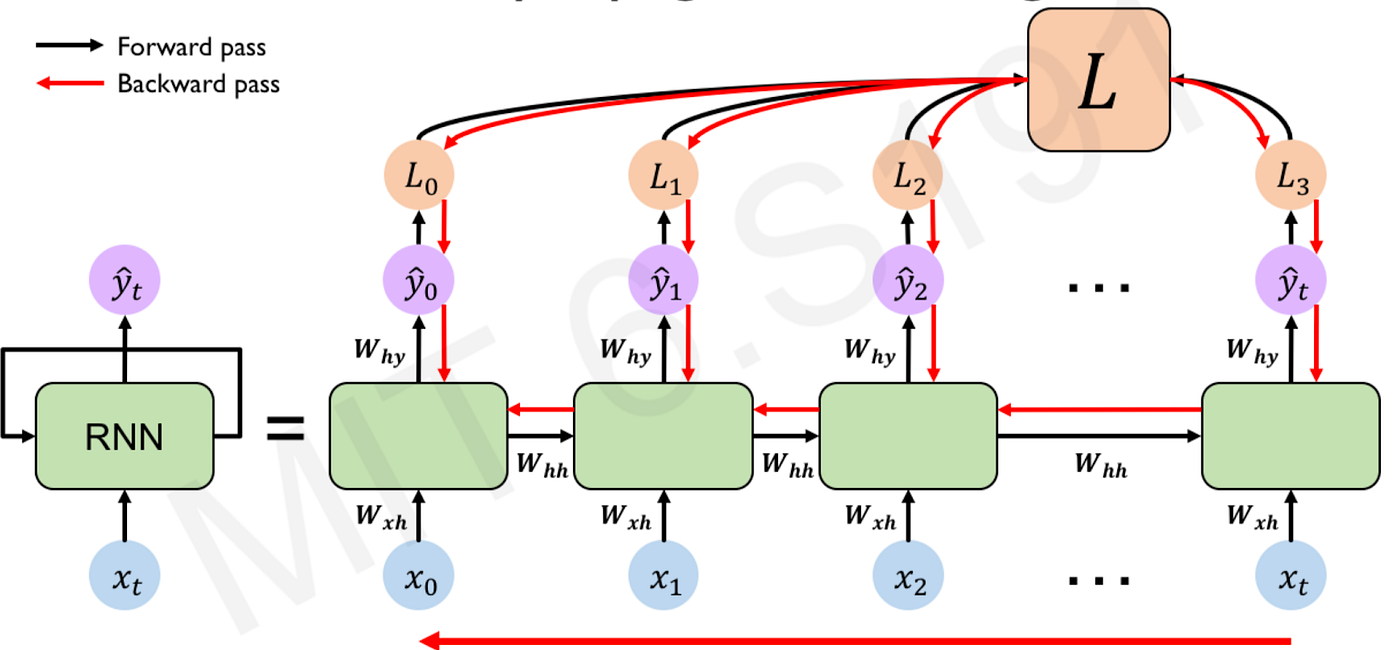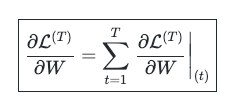Although RNNs learn contextual representations of sequential data, they suffer from the exploding and vanishing gradient phenomena in long sequences. These problems occur due to the multiplicative gradient that can exponentially increase or decrease through time. RNNs commonly use three activation functions: RELU, Tanh, and Sigmoid. Because the gradient calculation also involves the gradient with respect to the non-linear activations, architectures that use a RELU activation can suffer from the exploding gradient problem. Architectures that use Tanh/Sigmoid can suffer from the vanishing gradient problem. Gradient clipping — limiting the gradient within a specific range — can be used to remedy the exploding gradient. However, for the vanishing gradient problem, a more complex recurrent unit with gates such as Gated Recurrent Unit (GRU) or Long Short-Term Memory (LSTM) can be used.

#ai #recurrent-neural-network #attention-network #machine-learning #neural-network1599202560

Text Classification on Disaster Tweets with LSTM and Word Embedding

This was my first Kaggle notebook and I thought why not write it on Medium too?

Full code on my Github.

In this post, I will elaborate on how to use fastText and GloVe as word embedding on LSTM model for text classification. I got interested in Word Embedding while doing my paper on Natural Language Generation. It showed that embedding matrix for the weight on embedding layer improved the performance of the model. But since it was NLG, the measurement was objective. And I only used fastText too. So in this article, I want to see how each method (with fastText and GloVe and without) affects to the prediction. On my Github code, I also compare the result with CNN. The dataset that i use here is from one of competition on Kaggle, consisted of tweets and labelled with whether the tweet is using disastrous words to inform a real disaster or merely just used it metaphorically. Honestly, on first seeing this dataset, I immediately thought about BERT and its ability to understand way better than what I proposed on this article (further reading on BERT).

But anyway, in this article I will focus on fastText and GloVe.

Let’s go?

Data + Pre-Processing

The data consisted of 7613 tweets (columns Text) with label (column Target) whether they were talking about a real disaster or not. With 3271 rows informing real disaster and 4342 rows informing not real disaster. The data shared on kaggle competition, and if you want to learn more about the data you can read it here.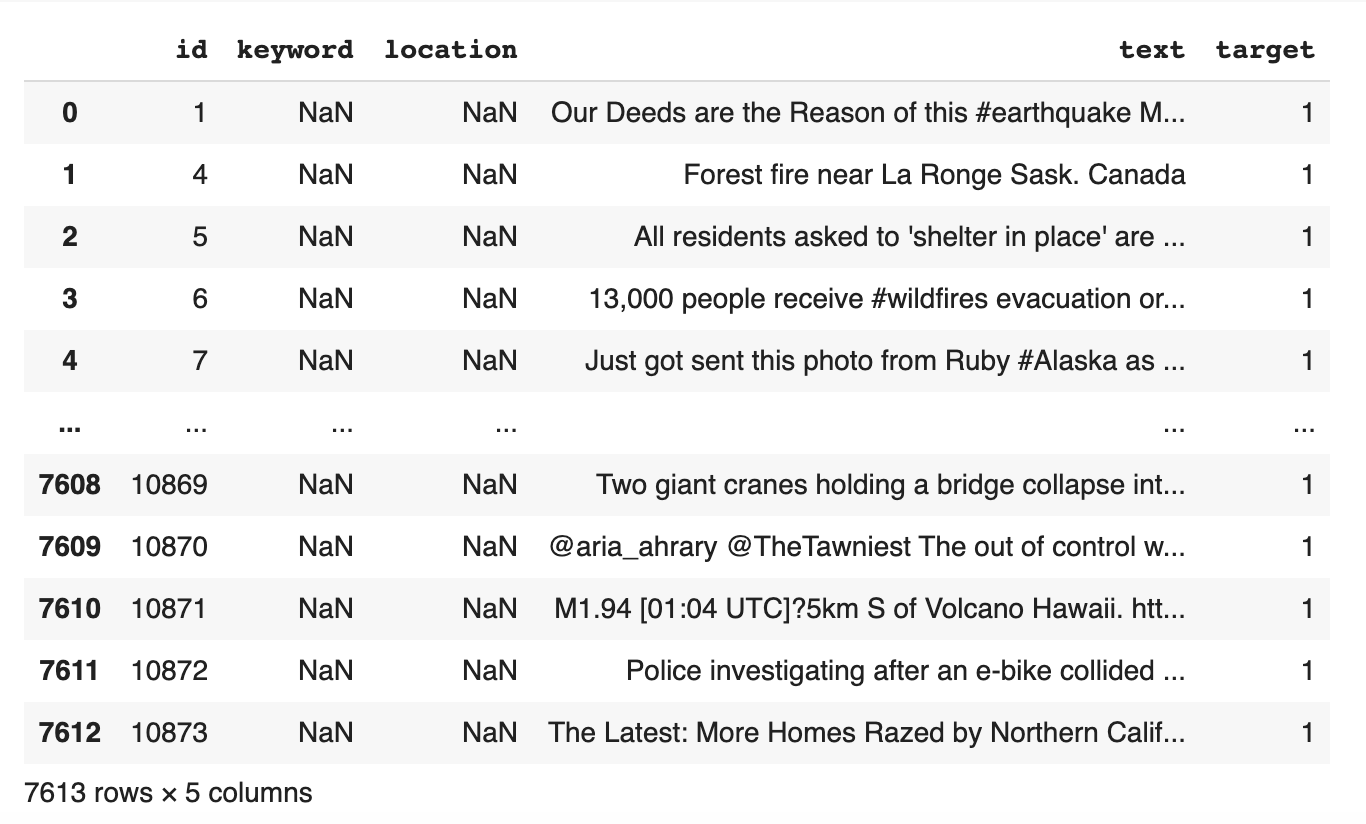Example of real disaster word in a text :

Example of the use of disaster word but not about disaster:

“These boxes are ready to explodeExploding Kittens finally arrived! gameofkittens #explodingkittens”

The data will be divided for training (6090 rows) and testing (1523 rows) then proceed to pre-processing. We will only be using the text and target columns.

#data-science #lstm #word-embeddings #nlp #text-classification #data analysis1596825840

A Comparative Analysis of Recurrent Neural Networks

Recurrent neural networks, also known as RNNs, are a class of neural networks that allow previous outputs to be used as inputs while having hidden states. RNN models are mostly used in the fields of natural language processing and speech recognition.

The vanishing and exploding gradient phenomena are often encountered in the context of RNNs. The reason why they happen is that it is difficult to capture long term dependencies because of multiplicative gradient that can be exponentially decreasing/increasing with respect to the number of layers.

Gated Recurrent Unit (GRU) and Long Short-Term Memory units (LSTM) deal with the vanishing gradient problem encountered by traditional RNNs, with LSTM being a generalization of GRU.

1D Convolution_ layer_ creates a convolution kernel that is convolved with the layer input over a single spatial (or temporal) dimension to produce a tensor of outputs. It is very effective for deriving features from a fixed-length segment of the overall dataset. A 1D CNN works well for natural language processing (NLP).

DATASET: IMDb Movie Review

TensorFlow Datasets is a collection of datasets ready to use, with TensorFlow or other Python ML frameworks, such as Jax. All datasets are exposed as [_tf.data.Datasets_](https://www.tensorflow.org/api_docs/python/tf/data/Dataset), enabling easy-to-use and high-performance input pipelines.

“imdb_reviews”

This is a dataset for binary sentiment classification containing substantially more data than previous benchmark datasets. It provides a set of 25,000 highly polar movie reviews for training, and 25,000 for testing.

Import Libraries

import pandas as pd
import numpy as np
import matplotlib.pyplot as plt
import seaborn as sns
%matplotlib inline

import tensorflow as tf
import tensorflow_datasets

imdb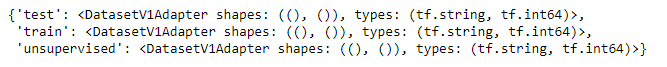info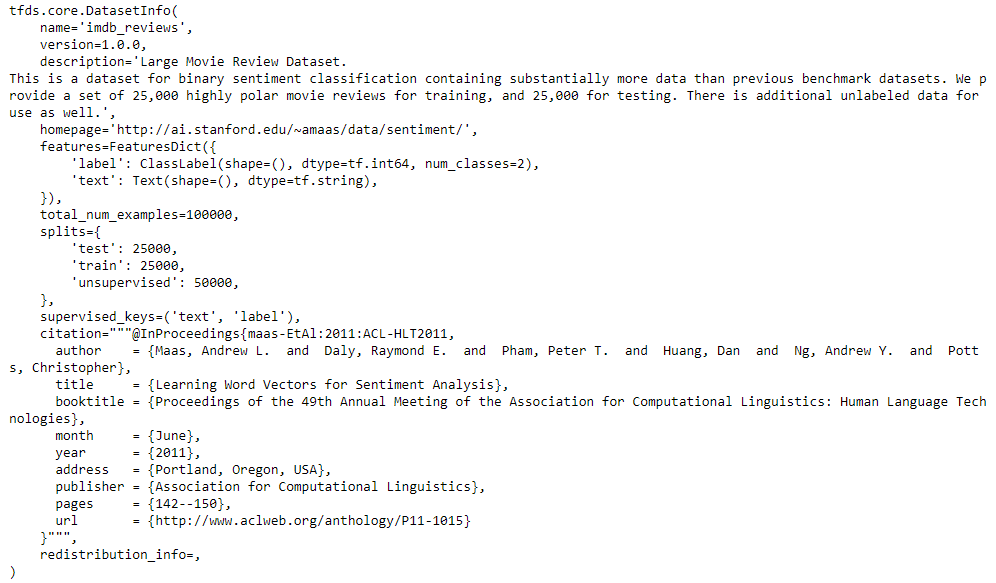Training and Testing Data

train_data, test_data=imdb['train'], imdb['test']

training_sentences=[]
training_label=[]
testing_sentences=[]
testing_label=[]
for s,l in train_data:
training_sentences.append(str(s.numpy()))
training_label.append(l.numpy())
for s,l in test_data:
testing_sentences.append(str(s.numpy()))
testing_label.append(l.numpy())
training_label_final=np.array(training_label)
testing_label_final=np.array(testing_label)

vocab_size=10000
embedding_dim=16
max_length=120
trunc_type='post'
oov_tok='<oov>'
from tensorflow.keras.preprocessing.text import Tokenizer